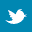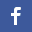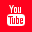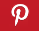Completely Solved C, C++ Programs Assignment.Quick Search Database Videos Tutorials Ebooks Forums FAQ Aboutus Household Industrial Manufacturing Service Shopping Transportation### C Program:While Loop

 Filed Under:

 While Loop:
Write a program in C to print the numbers 1 to 100
Write a program in C to sum of first 100 numbers
Write a program in C to sum of first N numbers
Write a program in C to sum of M to N numbers
Write a program in C to find the average of 1 to 100
Write a program in C to average of first N numbers
Write a program in C to average of M to N numbers
Write a program in C to display the even or odd numbers between 1 to 100
Write a program in C to display the even or odd numbers of first N numbers
Write a program in C to display the even or odd numbers between to ranges
Write a program in C to display the leap years between 2000 to 3000
Write a program in C to display the leap years of given range
Write a program in C to print the numbers between two ranges
Write a program in C to print the number until -1000 is given a input
Write a program in C to print the positive , negative and zero until -1000 is given a input
Write a program in C to count the positive, negative numbers and zero until -1000 is    given a input
Write a program in C to sum of the positive, negative numbers until -1000 is given a input
Write a program in C to mean of the positive , negative numbers until -1000 is given a input
Write a program in C to factorial of given number until -1000 is given a input
Write a program in C to find biggest of two numbers using ternary operator
Write a program in C to find smallest of two numbers using ternary operator
Write a program in C to find biggest of three numbers using ternary operator
Write a program in C to find smallest of three numbers using ternary operator
Write a program in C to find biggest of ten numbers
Write a program in C to find smallest of ten numbers
Write a program in C to find biggest of N numbers
Write a program in C to find smallest of N numbers using ternary operator
Write a program in C to read and display 10 numbers using array
Write a program in C to read and display of N numbers using array
Write a program in C to find mean of ten numbers using array
Write a program in C to find mean of N numbers using array
Write a program in C to find the biggest of 10 numbers using array
Write a program in C to find the smallest of 10 numbers using array
Write a program in C to find the position of biggest number in the array
Write a program in C to find the position of smallest number in the array
Write a program in C to find the positions of biggest&& smallest number in the array
Write a program in C to interchange the biggest & smallest number in the array
Write a program in C to find the second biggest of N numbers using array
Write a program in C to find the second smallest of N numbers using array
Write a program in C to find the second biggest and smallest of N numbers using array
Write a program in C to find the positions of second biggest and smallest of N numbers using array
Write a program in C to interchange the second biggest and smallest of N numbers using array